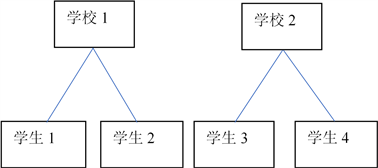# 多水平模型在中学生数学考试成绩影响因素分析中的应用Application of Multi-Level Model in the Analysis of Influencing Factors of Mathematics Examination in Middle School

DOI: 10.12677/ASS.2019.82028, PDF, HTML, XML, 下载: 448  浏览: 754  科研立项经费支持

Abstract: Education measurement and teaching evaluation is an important part of teaching activities. One of the main parts in teaching evaluation is the analysis of examination results and its influencing factors. At present, the main methods include descriptive analysis, cluster analysis, principal component analysis, factor analysis and multiple linear regression analysis. However, the data with hierarchical structure is not suitable to be analyzed by the above methods. Based on the sample data, a multi-level model is established. The results showed that school, gender and family were significantly affected by education.

1. 引言

2. 数据来源与研究方法

2.1. 数据来源与数据处理

2.2. 研究方法${y}_{ij}={\beta }_{oj}+{\beta }_{1}{X}_{ij}+{e}_{ij}$ (1)

${\beta }_{0j}={\gamma }_{00}+{\mu }_{0j}$ (2)

${y}_{ij}$${X}_{ij}$ 分别为第j个学校中第i个学生应变量观测值和解释变量观测值。 ${\gamma }_{00}$${\beta }_{0j}$ 的平均值，为固定成份， ${\mu }_{0j}$ 为水平2上( ${\beta }_{0j}$ )的随机成分，服从正态分布。 ${e}_{ij}$ 表示水平1上的随机误差，也服从正态分布。把(2)式代入(1)式并整理，得：

${y}_{ij}=\left({\gamma }_{00}+{\beta }_{1}{X}_{ij}\right)+\left({\mu }_{0j}+{e}_{ij}\right)$ (3)

$Var\left({y}_{ij}|{\gamma }_{00},{\beta }_{1},{X}_{ij}\right)=Var\left({\mu }_{0j}+{e}_{0ij}\right)=Var\left({\mu }_{0j}\right)+Var\left({e}_{0ij}\right)+Cov\left({\mu }_{0j},{e}_{0ij}\right)={\sigma }_{{\mu }_{0}}^{2}+{\sigma }_{{e}_{0}}^{2}$

$Cov\left({\mu }_{0j}+{e}_{{i}_{1}j},{\mu }_{0j}+{e}_{{i}_{2}j}\right)=Cov\left({\mu }_{0j},{\mu }_{0j}\right)={\sigma }_{{\mu }_{0}}^{2}$

$\rho =\frac{{\sigma }_{{\mu }_{0}}^{2}}{{\sigma }_{{\mu }_{0}}^{2}+{\sigma }_{{e}_{0}}^{2}}$ (4)

ICC测量了学校间方差占总方差的比例，实际上它反映了学校内个体间相关，即水平1单位(学生)在水平2单位(学校)中的聚集性或相似性。由于模型不止一个残差项，就产生了非零的组内相关。若ICC为0，表明数据不具备层次结构，可忽略学校的存在，即转化为传统的单水平模型；反之，若存在非零的 ${\sigma }_{{\mu }_{0}}^{2}$ ，则不能忽略学校的存在。

3. 结果分析

3.1. 模型一：无解释变量(空模型)Table 1. Model test results when no explanatory variables are assumed

3.2. 模型二：引入变量Table 2. Model test results after introducing gender variables

3.3. 模型三：引入家庭受教育程度变量Table 3. Model test results after introducing gender and education variables

4. 结论

  王艳梅. 多水平模型在大学英语考试成绩影响因素分析中的应用[D]: [硕士学位论文]. 济南: 山东大学, 2007.  陈光慧, 刘建平. 我国基本单位多水平连续性抽样调查体系改革研究[J]. 统计研究, 2014, 31(7): 50-57.  麻吉亮, 陈永福, 钱小平. 气候因素、中间投入与玉米单产增长——基于河北农户层面多水平模型的实证分析[J]. 中国农村经济, 2012(11): 11-20.  石磊, 向其凤. 鲁筠. 多水平模型及其在经济分析中的应用[J]. 数学建模及其应用, 2016, 5(1): 3-8.  谢传波, 牛中正, 丁鹏, 等. 多水平统计模型的Stata程序实现[J]. 中国卫生统计, 2014, 31(1): 169-170.  赵金辉, 魏建荣, 陈华洁, 等. 北京地区居民日均饮水量相关因素的多水平模型分析[J]. 卫生研究, 2012, 41(5): 809-813.  梁宗保, 张光珍, 邓慧华, 等. 学前儿童努力控制发展轨迹与父母养育的关系[J]. 心理学报, 2013, 45(5): 556-567.  康春花, 孙小坚, 顾士伟, 等. 多水平多维IRT模型在学业质量监测中的应用[J]. 江西师范大学学报(自然科学版), 2016, 40(2): 133-139.